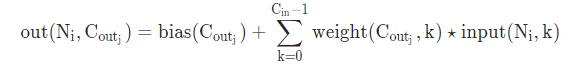# Does Conv2d filter need bias per weights?

Let’s say I have an image with (3,32,32) size and I want to apply (32,4,4) filter.
If I use “torch.nn.Conv2d” thenby this formula, I have 32 bias values.
If let’s say adding bias at each weight, then I’ll have 32x(4x4)= 512 bias values.
Is there any reason not applying bias terms at each weight in the Conv2d filter?

The filter will be applied as an elementwise multiplication to the current input window and summed afterwards to create a scalar output value. The bias will then be added to the scalar value, which explains the shape.